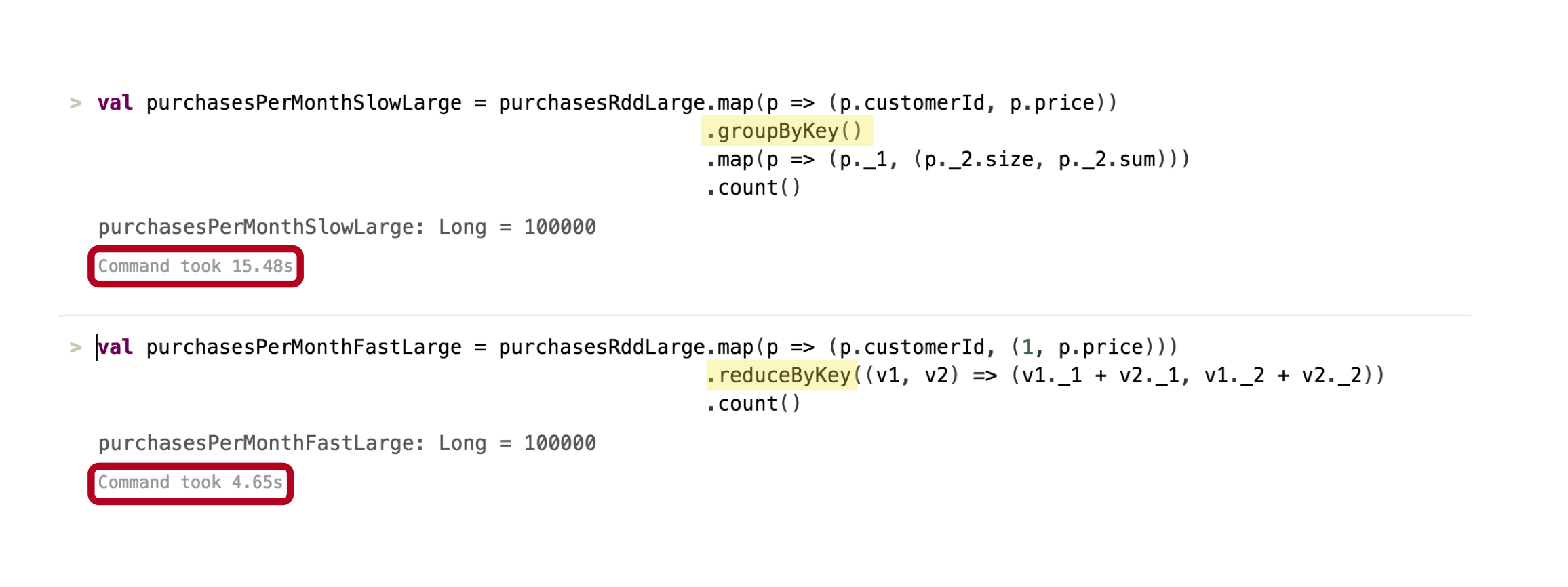# Programmation appliquée en Scala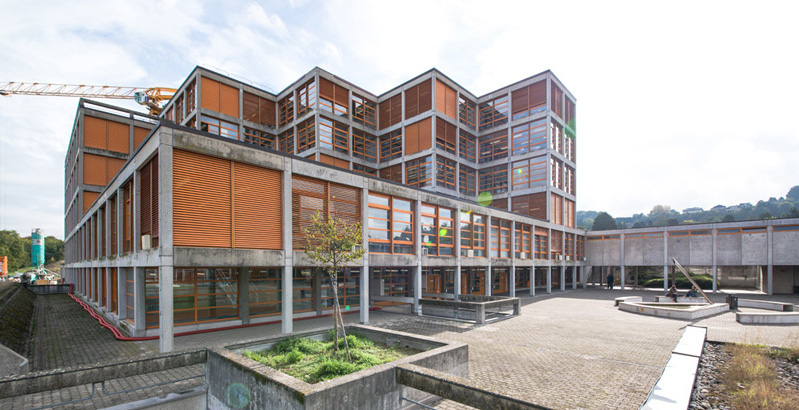Copyright © Cay S. Horstmann 2015# What we’ve seen so far

Note: The content of this unit is adapted from the slides of the course "Parallel Programming and Data Analysis"
by Heather Miller at EPFL.

Spark’s Programming Model

• We saw that, at a glance, Spark looks like Scala collections
• However, interally, Spark behaves differently than Scala collections
• Spark uses laziness to save time and memory
• We saw transformations and actions
• We saw caching and persistence (i.e., cache in memory, save time!)
• We saw how the cluster topology comes into the programming model
• We got a sampling of Spark’s key-value pairs (Pair RDDs)

# In this unit...

1. Reduction operations in Spark vs Scala collections
2. More on Pair RDDs (key-value pairs)
3. We’ll get a glimpse of what “shuffling” is, and why it hits performance (latency)

# Reduction Operations

Spark provides a subset of the reduction operations of Scala.
In fact, not all reduction operations are parallelizable.

Take the two operations, fold and foldLeft. Which of these two are parallelizable?

def foldLeft[B](z: B)(f: (B, A) => B): B


def fold(z: A)(f: (A, A) => A): A

# Non-Parallelizable Operations

Spark provides a subset of the reduction operations of Scala.
In fact, not all reduction operations are parralizable.

Let's examine the foldLeft signature.

def foldLeft[B](z: B)(f: (B, A) => B): B# Non-Parallelizable Operations

Spark provides a subset of the reduction operations of Scala.
In fact, not all reduction operations are parrallelizable.

Let's examine the foldLeft signature.

def foldLeft[B](z: B)(f: (B, A) => B): B


# Non-Parallelizable Operations

Being able to change the result type from A to B forces us to have to
execute foldLeft sequentially from left to right.

Concretely, given:

val xs = List(1, 2, 3)
val res = xs.foldLeft("")((str: String, i: Int) => str + i)


What happens if we try to break this collection in two and parallelize?foldLeft is not parallelizable.

Operations foldRight, reduceLeft, reduceRight, scanLeft and scanRight
similarly must process elements sequentially.

# Reduction Operations: Fold

fold enables us to parallelize things, but it restricts us to always
returning the same type.

def fold(z: A)(f: (A, A) => A): A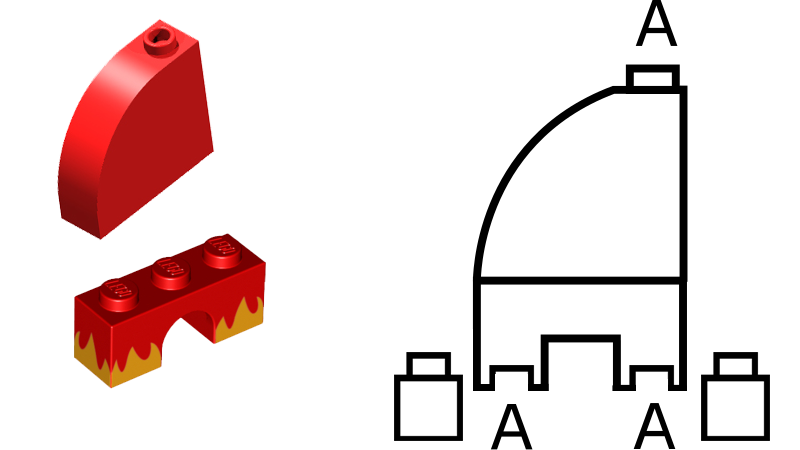**It enables us to parallelize using a single function f by enabling us to
build parallelizable reduce trees.**

# Reduction Operations: Fold

**It enables us to parallelize using a single function f by enabling us to
build parallelizable reduce trees.**

def fold(z: A)(f: (A, A) => A): A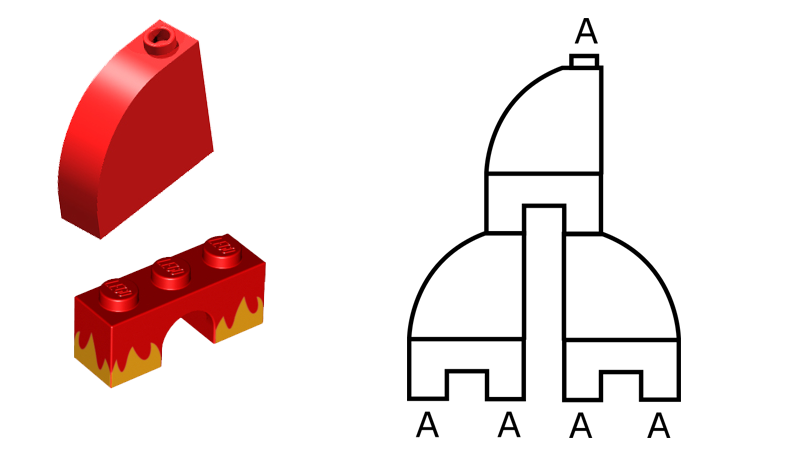# Reduction Operations: Aggregate

Has someone already used the aggregate operation?

aggregate[B](z: => B)(seqop: (B, A) => B, combop: (B, B) => B): B


aggregate is said to be general because it gets you the best of both
worlds.

Properties of aggregate

1. Parallelizable.
2. Possible to change the return type.

# Reduction Operations: Aggregate

aggregate[B](z: => B)(seqop: (B, A) => B, combop: (B, B) => B): BAggregate lets you still do sequential-style folds in chunks which change
the result type. Additionally requiring the combop function enables building
one of these nice reduce trees that we saw is possible with fold to combine
these chunks in parallel.

# Reduction Operations on RDDs

 Scala collections: Spark: fold fold foldLeft/foldRight - reduce reduce aggregate aggregate

Spark doesn’t even give you the option to use foldLeft/foldRight. Which
means that if you have to change the return type of your reduction operation,
your only choice is to use aggregate.

# RDD Reduction Operations: Aggregate

In Spark, aggregate is a more desirable reduction operator a majority of the
time.

As you will realize from experimenting with Spark , much of the time when working with large-scale data, our goal is to project down from larger/more complex data types

Example:

case class WikipediaPage(
title: String,
redirectTitle: String,
timestamp: String,
text: String)


We might only care about title and timestamp, for example. In this case, it’d save a lot of time/memory
to not have to carry around the full-text of each article in
our accumulator!

# Pair RDDs (Key-Value Pairs)

Key-value pairs are known as Pair RDDs in Spark.

When an RDD is created with a pair as its element type, Spark automatically
adds a number of extra useful additional methods (extension methods) for such
pairs.

# Pair RDDs (Key-Value Pairs)

Creating a Pair RDD

Pair RDDs are most often created from already-existing non-pair RDDs,
for example by using the map operation on RDDs:

val rdd: RDD[WikipediaPage] = ...

val pairRdd = ???


# Pair RDDs (Key-Value Pairs)

Creating a Pair RDD

Pair RDDs are most often created from already-existing non-pair RDDs,
for example by using the map operation on RDDs:

val rdd: RDD[WikipediaPage] = ...

// Has type: org.apache.spark.rdd.RDD[(String, String)]
val pairRdd = rdd.map(page => (page.title, page.text))


Once created, you can now use transformations specific to key-value pairs such
as reduceByKey, groupByKey, and join

# Some interesting Pair RDDs operations

Transformations

• groupByKey
• reduceByKey
• join
• leftOuterJoin/rightOuterJoin

Action

• countByKey

# Pair RDD Transformation: groupByKey

Recall groupBy from Scala collections. groupByKey can be thought of as a
groupBy on Pair RDDs that is specialized on grouping all values that have
the same key. As a result, it takes no argument.

def groupByKey(): RDD[(K, Iterable[V])]


Example:

case class Event(organizer: String, name: String, budget: Int)
val eventsRdd = sc.parallelize(...)
.map(event => (event.organizer, event.budget))

val groupedRdd = eventsRdd.groupByKey()


Here the key is organizer. What does this call do?

# Pair RDD Transformation: groupByKey

Example:

case class Event(organizer: String, name: String, budget: Int)
val eventsRdd = sc.parallelize(...)
.map(event => (event.organizer, event.budget))

val groupedRdd = eventsRdd.groupByKey()
groupedRdd.collect().foreach(println)
// (Prime Sound,CompactBuffer(42000))
// (Sportorg,CompactBuffer(23000, 12000, 1400))
// ...


# Pair RDD Transformation: reduceByKey

Conceptually, reduceByKey can be thought of as a combination of groupByKey
and reduce-ing on all the values per key. It’s more efficient though, than
using each separately. (We’ll see why later.)

def reduceByKey(func: (V, V) => V): RDD[(K, V)]


Example: Let’s use eventsRdd from the previous example to calculate the
total budget per organizer of all of their organized events.

case class Event(organizer: String, name: String, budget: Int)
val eventsRdd = sc.parallelize(...)
.map(event => (event.organizer, event.budget))

val budgetsRdd = ...


# Pair RDD Transformation: reduceByKey

Example: Let’s use eventsRdd from the previous example to calculate the
total budget per organizer of all of their organized events.

case class Event(organizer: String, name: String, budget: Int)
val eventsRdd = sc.parallelize(...)
.map(event => (event.organizer, event.budget))

val budgetsRdd = eventsRdd.reduceByKey(_+_)

reducedRdd.collect().foreach(println)
// (Prime Sound,42000)
// (Sportorg,36400)
// (Innotech,320000)
// (Association Balélec,50000)


# Pair RDD Transformation: mapValues and Action: countByKey

def mapValues[U](f: (V) ⇒ U): RDD[(K, U)]

mapValues can be thought of as a short-hand for:

rdd.map { case (x, y): (x, func(y))}


That is, it simply applies a function to only the values in a Pair RDD.

countByKey (def countByKey(): Map[K, Long]) simply counts the number
of elements per key in a Pair RDD, returning a normal Scala Map (remember,
it’s an action!) mapping from keys to counts.

# Pair RDD Transformation: mapValues and Action: countByKey

Example: we can use each of these operations to compute the average budget
per event organizer.

// Calculate a pair (as a key's value) containing (budget, #events)
val intermediate =
eventsRdd.mapValues(b => (b, 1))
.reduceByKey((v1, v2) => (v1._1 + v2._1, v1._2 + v2._2))
// intermediate: RDD[(String, (Int, Int))]

val avgBudgets = ???


# Pair RDD Transformation: mapValues and Action: countByKey

Example: we can use each of these operations to compute the average budget
per event organizer.

// Calculate a pair (as a key's value) containing (budget, #events)
val intermediate =
eventsRdd.mapValues(b => (b, 1))
.reduceByKey((v1, v2) => (v1._1 + v2._1, v1._2 + v2._2))
// intermediate: RDD[(String, (Int, Int))]

val avgBudgets = intermediate.mapValues {
case (budget, numberOfEvents) => budget / numberOfEvents
}
avgBudgets.collect().foreach(println)
// (Prime Sound,42000)
// (Sportorg,12133)
// (Innotech,106666)
// (Association Balélec,50000)


# Joins

Joins are another sort of transformation on Pair RDDs. They’re used to combine
multiple datasets They are one of the most commonly-used operations on Pair
RDDs!

There are two kinds of joins:

• Inner joins (join)
• Outer joins (leftOuterJoin/rightOuterJoin)

The difference between the two types of joins is exactly the same as in databases

# Inner Joins (join)

Inner joins return a new RDD containing combined pairs whose keys are present in both input RDDs.

def join[W](other: RDD[(K, W)]): RDD[(K, (V, W))]


Example: Let’s pretend the CFF has two datasets. One RDD representing
customers and their subscriptions (abos), and another representing
customers and cities they frequently travel to (locations). (E.g.,
gathered from CFF smartphone app.)

How do we combine only customers that have a subscription and where there is
location info?

val abos = ... // RDD[(Int, (String, Abonnement))]
val locations = ... // RDD[(Int, String)]

val trackedCustomers = ???


# Inner Joins (join)

Example: Let’s pretend the CFF has two datasets. One RDD representing
customers and their subscriptions (abos), and another representing
customers and cities they frequently travel to (locations). (E.g.,
gathered from CFF smartphone app.)

How do we combine only customers that have a subscription and where there is
location info?

val abos = ... // RDD[(Int, (String, Abonnement))]
val locations = ... // RDD[(Int, String)]

val trackedCustomers = abos.join(locations)
// trackedCustomers: RDD[(Int, ((String, Abonnement), String))]


# Inner Joins (join)

Example continued with concrete data:

val as = List((101, ("Ruetli", AG)), (102, ("Brelaz", DemiTarif)),
(103, ("Gress", DemiTarifVisa)), (104, ("Schatten", DemiTarif)))
val abos = sc.parallelize(as)

val ls = List((101, "Bern"), (101, "Thun"), (102, "Lausanne"), (102, "Geneve"),
(102, "Nyon"), (103, "Zurich"), (103, "St-Gallen"), (103, "Chur"))
vals locations = sc.parallelize(ls)

val trackedCustomers = abos.join(locations)
// trackedCustomers: RDD[(Int, ((String, Abonnement), String))]


# Inner Joins (join)

Example continued with concrete data:

trackedCustomers.collect().foreach(println)
// (101,((Ruetli,AG),Bern))
// (101,((Ruetli,AG),Thun))
// (102,((Brelaz,DemiTarif),Nyon))
// (102,((Brelaz,DemiTarif),Lausanne))
// (102,((Brelaz,DemiTarif),Geneve))
// (103,((Gress,DemiTarifVisa),St-Gallen))
// (103,((Gress,DemiTarifVisa),Chur))
// (103,((Gress,DemiTarifVisa),Zurich))


What happened to customer 104?

# Resources to learn more operations on Pair RDDs

Book

The Learning Spark book is the most complete reference.

Free

• Spark Programming Guide
Contains compact overview of Spark's programming model. Lists table of all transformers vs accessors, for example. However, doesn't go into a lot of detail about individual operations.
• Spark API Docs
Look for class PairRDDFunctions for all possible operations on Pair RDDs.

# Optimizing...

Now that we understand Spark’s programming model, and a majority of Spark’s
key operations, we’ll now see how we can optimize what we do with Spark to
keep it practical.

It’s very easy to write clear code that takes tens of minutes to compute when
it could be computed in only tends of seconds!

# Grouping and Reducing, Example

case class CFFPurchase(customerId: Int, destination: String, price: Double)


Assume we have an RDD of the purchases that users of the CFF mobile app have
made in the past month.

val purchasesRdd: RDD[CFFPurchase] = sc.textFile(...)


Goal: calculate how many trips, and how much money
was spent by each individual customer over the course of the month.

# Grouping and Reducing, Example

**Goal: calculate how many trips, and how much money was spent by each
individual customer over the course of the month.**

val purchasesRdd: RDD[CFFPurchase] = sc.textFile(...)

val purchasesPerMonth = ...


# Grouping and Reducing, Example

**Goal: calculate how many trips, and how much money was spent by each
individual customer over the course of the month.**

val purchasesRdd: RDD[CFFPurchase] = sc.textFile(...)

val purchasesPerMonth =
purchasesRdd.map(p => (p.customerId, p.price)) // Pair RDD


# Grouping and Reducing, Example

**Goal: calculate how many trips, and how much money was spent by each
individual customer over the course of the month.**

val purchasesRdd: RDD[CFFPurchase] = sc.textFile(...)

val purchasesPerMonth =
purchasesRdd.map(p => (p.customerId, p.price)) // Pair RDD
.groupByKey() // groupByKey returns RDD[K, Iterable[V]]


# Grouping and Reducing, Example

**Goal: calculate how many trips, and how much money was spent by each
individual customer over the course of the month.**

val purchasesRdd: RDD[CFFPurchase] = sc.textFile(...)

// Returns: Array[(Int, (Int, Double))]
val purchasesPerMonth =
purchasesRdd.map(p => (p.customerId, p.price)) // Pair RDD
.groupByKey() // groupByKey returns RDD[K, Iterable[V]]
.map(p => (p._1, (p._2.size, p._2.sum)))
.collect()


# Grouping and Reducing, Example – What’s Happening?

val purchases = List(CFFPurchase(100, "Geneva", 22.25),
CFFPurchase(300, "Zurich", 42.10),
CFFPurchase(100, "Fribourg", 12.40),
CFFPurchase(200, "St. Gallen", 8.20),
CFFPurchase(100, "Lucerne", 31.60),
CFFPurchase(300, "Basel", 16.20))


What might the cluster look like with this data distributed over it?

# Grouping and Reducing, Example – What’s Happening?

What might the cluster look like with this data distributed over it?

Starting with purchasesRdd: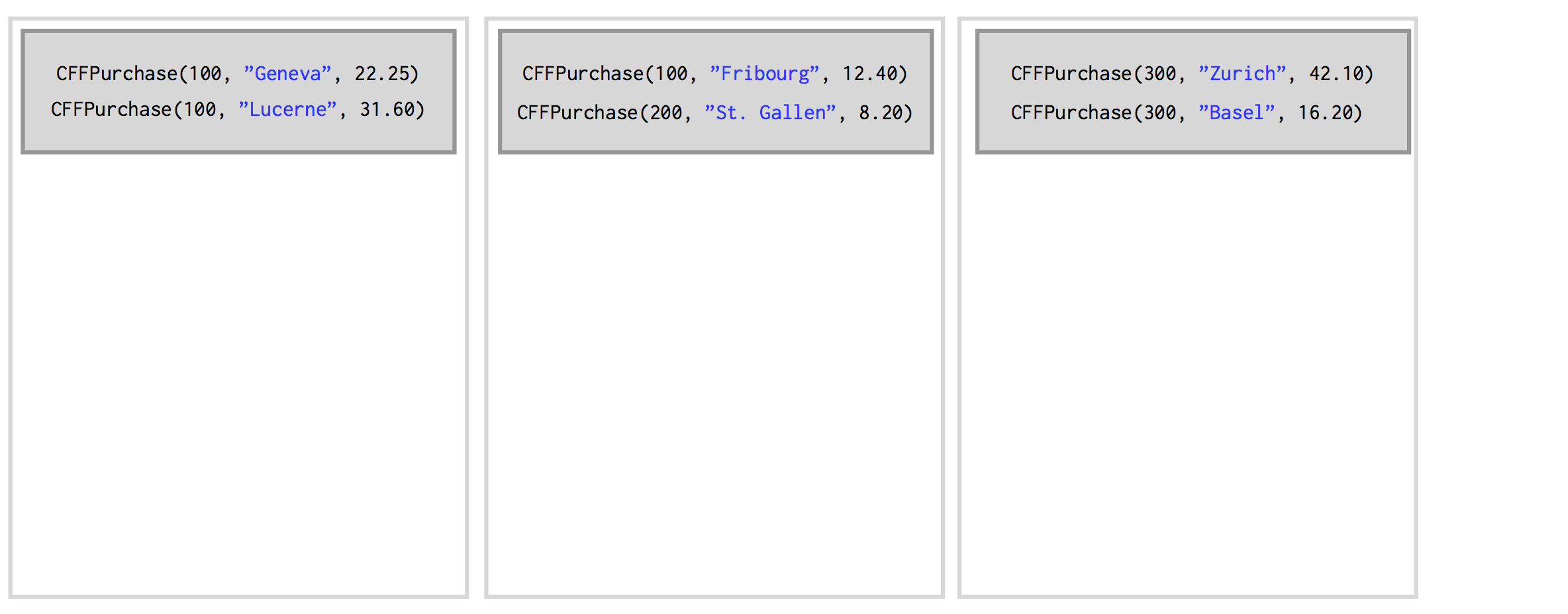# Grouping and Reducing, Example – What’s Happening?

What might this look like on the cluster?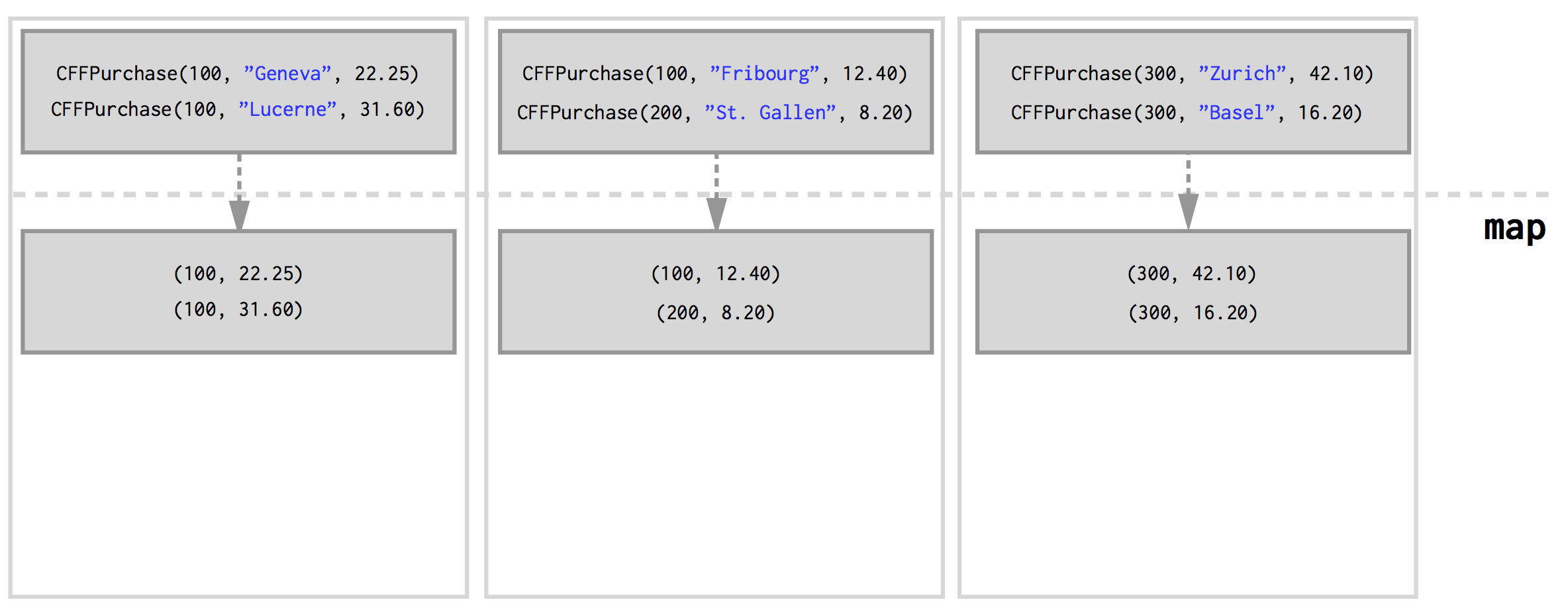# Grouping and Reducing, Example

**Goal: calculate how many trips, and how much money was spent by each
individual customer over the course of the month.**

val purchasesRdd: RDD[CFFPurchase] = sc.textFile(...)

val purchasesPerMonth =
purchasesRdd.map(p => (p.customerId, p.price)) // Pair RDD
.groupByKey() // groupByKey returns RDD[K, Iterable[V]]


Note: groupByKey results in one key-value pair per key.
And this single key-value pair cannot span across multiple worker nodes.

# Grouping and Reducing, Example – What’s Happening?

What might this look like on the cluster?# Grouping and Reducing, Example – What’s Happening?

What might this look like on the cluster?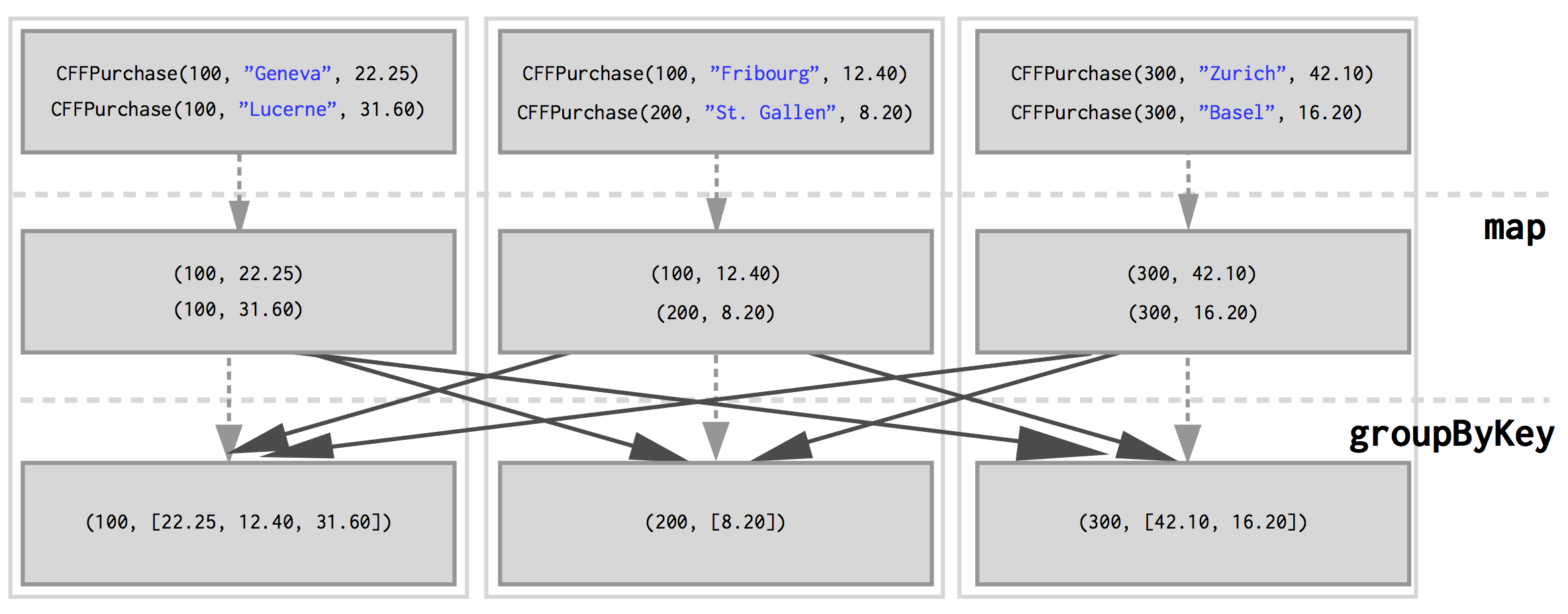# Grouping and Reducing, Example – What’s Happening?

What might this look like on the cluster?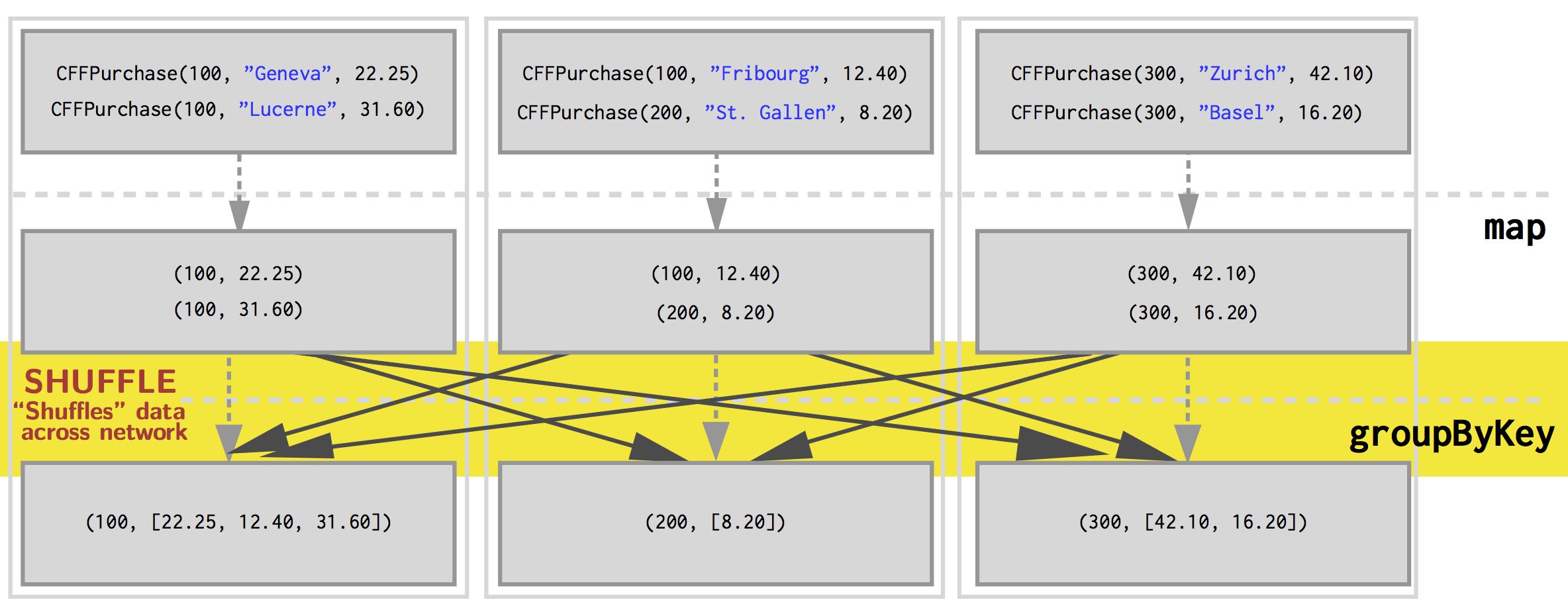But, we don’t want to be sending all of our data over the network if it’s not absolutely required.
Too much network communication kills performance.

# Can we do a better job?

Perhaps we don’t need to send all pairs over the network.Perhaps we can reduce before we shuffle. This could greatly reduce the amount of data we have to send over the network.

# Grouping and Reducing, Example – Optimized

We can use reduceByKey.

Conceptually, reduceByKey can be thought of as a combination of first doing
groupByKey and then reduce-ing on all the values grouped per key. It’s
more efficient though, than using each separately. We’ll see how in the
following example.

Signature:

def reduceByKey(func: (V, V) => V): RDD[(K, V)]


# Grouping and Reducing, Example – Optimized

Goal: calculate how many trips, and how much money was spent by each
individual customer over the course of the month.

val purchasesRdd: RDD[CFFPurchase] = sc.textFile(...)

val purchasesPerMonth =
purchasesRdd.map(p => (p.customerId, (1, p.price))) // Pair RDD
.reduceByKey(...) // ?


Notice that the function passed to map has changed.
It’s now p => (p.customerId, (1, p.price))

**What function do we pass to reduceByKey in order to get a result that
looks like: (customerId, (numTrips, totalSpent)) returned?**

# Grouping and Reducing, Example – Optimized

val purchasesPerMonth =
purchasesRdd.map(p => (p.customerId, (1, p.price))) // Pair RDD
.reduceByKey(...) // ?


Recall that we’re reducing over the values per key.

Since our values are an Iterable[(Int, Double)], the function that we pass
to reduceByKey must reduce over two such pairs.

# Grouping and Reducing, Example – Optimized

val purchasesPerMonth =
purchasesRdd.map(p => (p.customerId, (1, p.price))) // Pair RDD
.reduceByKey((v1, v2) => (v1._1 + v2._1, v1._2 + v2._2))
.collect()


What might this look like on the cluster?

# Grouping and Reducing, Example – Optimized

What might this look like on the cluster?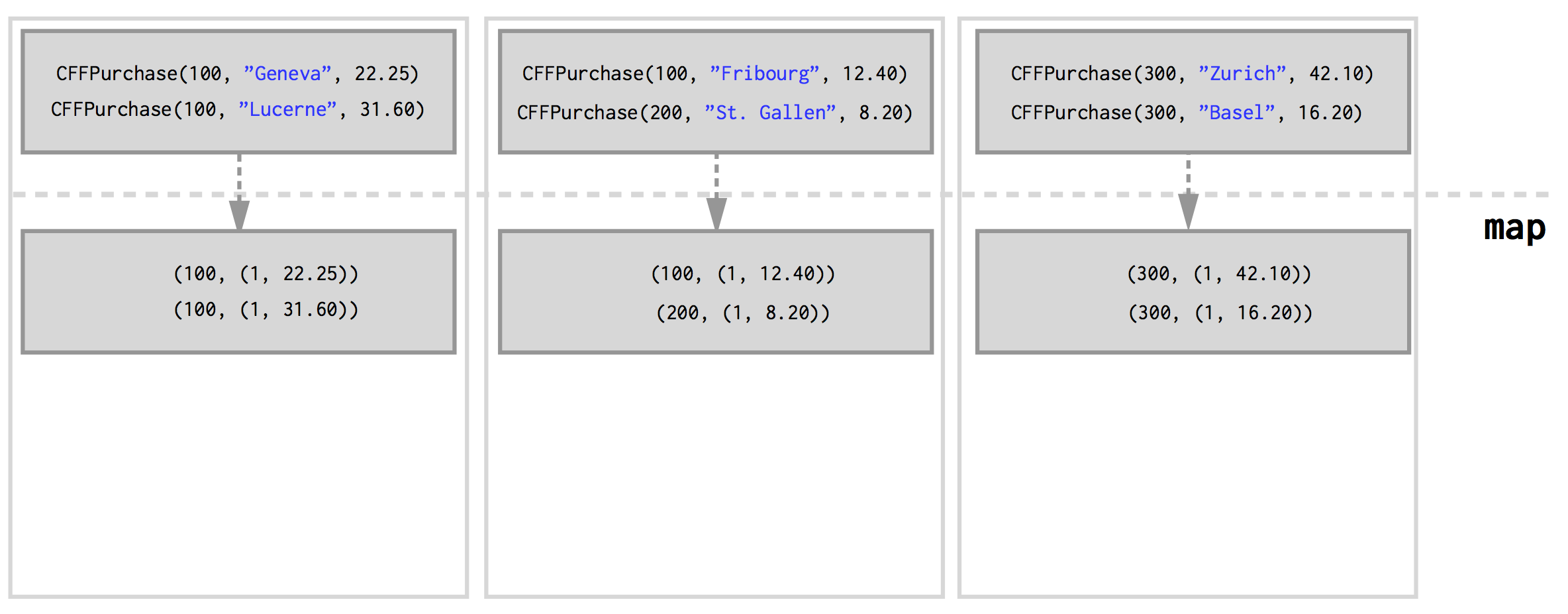# Grouping and Reducing, Example – Optimized

What might this look like on the cluster?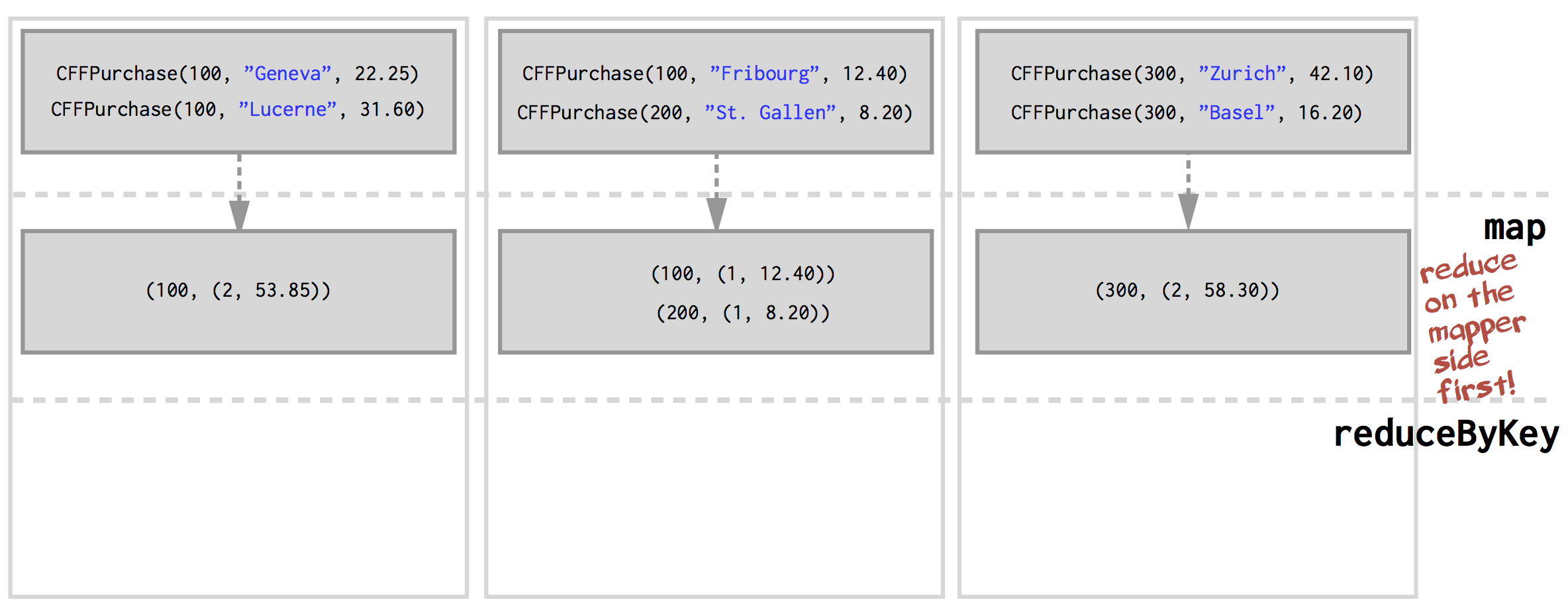# Grouping and Reducing, Example – Optimized

What might this look like on the cluster?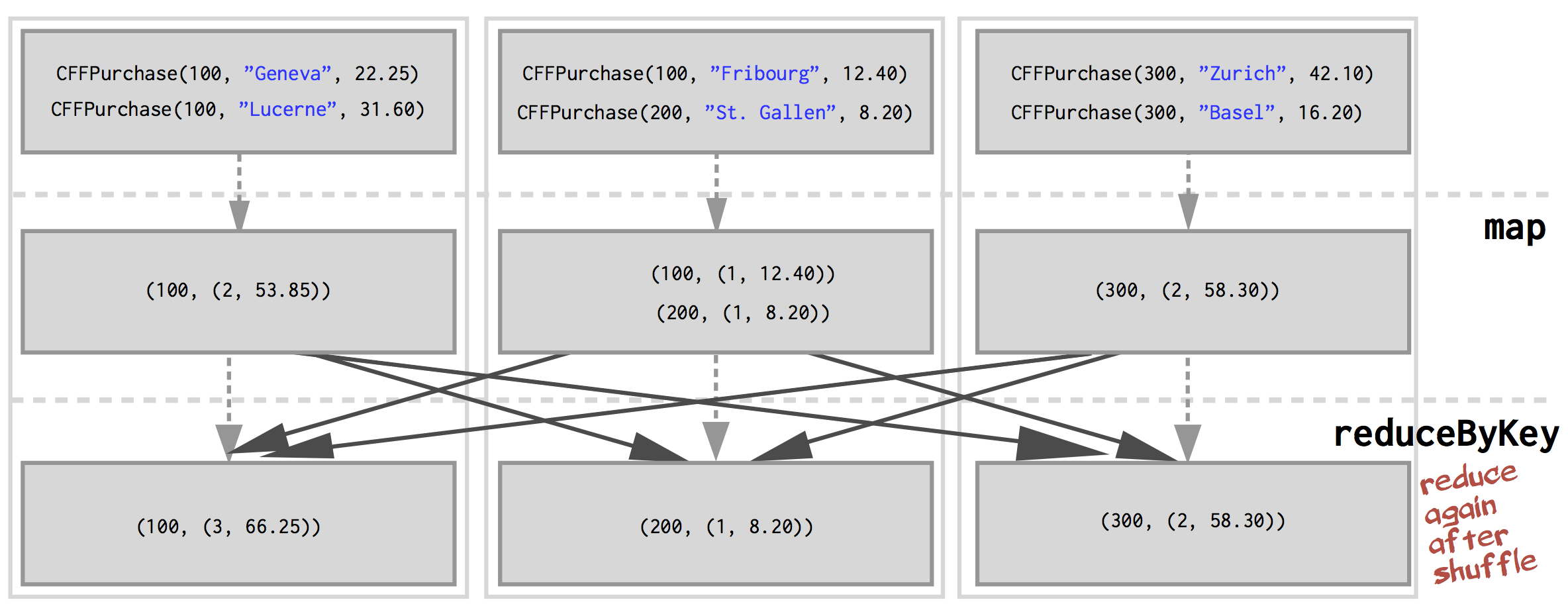# Grouping and Reducing, Example – Optimized

What are the benefits of this approach?

By reducing the dataset first, the amount of data sent over the network during
the shuffle is greatly reduced.

This can result in non-trival gains in performance!

# groupByKey and reduceByKey Running Times

Benchmark results on a real cluster: